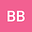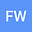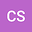loading page

The Eigenspace Spectral Regularization Method for solving Discrete Ill-Posed Systems
•••• +1FREDRICK WIREKO
Kwame Nkrumah University of Science and Technology
Author ProfileCharles Sebil;
Kwame Nkrumah University of Science and Technology
Author ProfileJoseph Ackora-Prah
Kwame Nkrumah University of Science and Technology
Author Profile## Abstract

In this paper, it is shown that discrete equations with Hilb ert matrix operator, circulant matrix operator, conference matrix operator, banded matrix operator, and sparse matrix operator are ill-posed in the sense of Hadamard. These ill-posed problems cannot be regularized by Gauss Least Square Method (GLSM), QR Factorization Method (QRFM), Cholesky Decomposition Method (CDM) and Singular Value Decomposition (SVDM). To overcome the limitations of these methods of regularization, an Eigenspace Spectral Regularization Method (ESRM) is introduced which solves ill-p os ed discrete equations with Hilb ert matrix operator, circulant matrix operator, conference matrix operator, banded matrix operator, and sparse matrix operator. Unlike GLSM, QRFM, CDM, and SVDM, the ESRM regularize such a system. In addition, the ESRM has a unique property, the norm of the eigenspace spectral matrix operator κ (K) = ||K − 1K|| = 1. Thus, the condition number of ESRM is bounded by unity unlike the other regularization methods such as SVDM, GLSM, CDM, and QRFM.